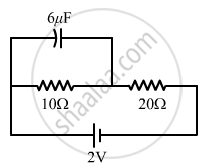Share

# Find the Charge on the Capacitor as Shown in the Circuit. - Physics

ConceptEnergy Stored in a Capacitor

#### Question

Find the charge on the capacitor as shown in the circuit.#### Solution

Total current I through the circuit is given by

$I = \frac{V}{R}$
Here, V = 2 V
= (10+20) Ω = 30 Ω
$\therefore I = \frac{2}{30} = \frac{1}{15} A$
Voltage across 10 Ω resistor =$I(10) = \frac{10}{15} = \frac{2}{3} V$
∴ Charge on the capacitor is given by $Q = CV = \left( 6 \times {10}^{- 6} \right)\left( \frac{2}{3} \right) = 4 \mu C$
Is there an error in this question or solution?

#### Video TutorialsVIEW ALL 

Solution Find the Charge on the Capacitor as Shown in the Circuit. Concept: Energy Stored in a Capacitor.
S